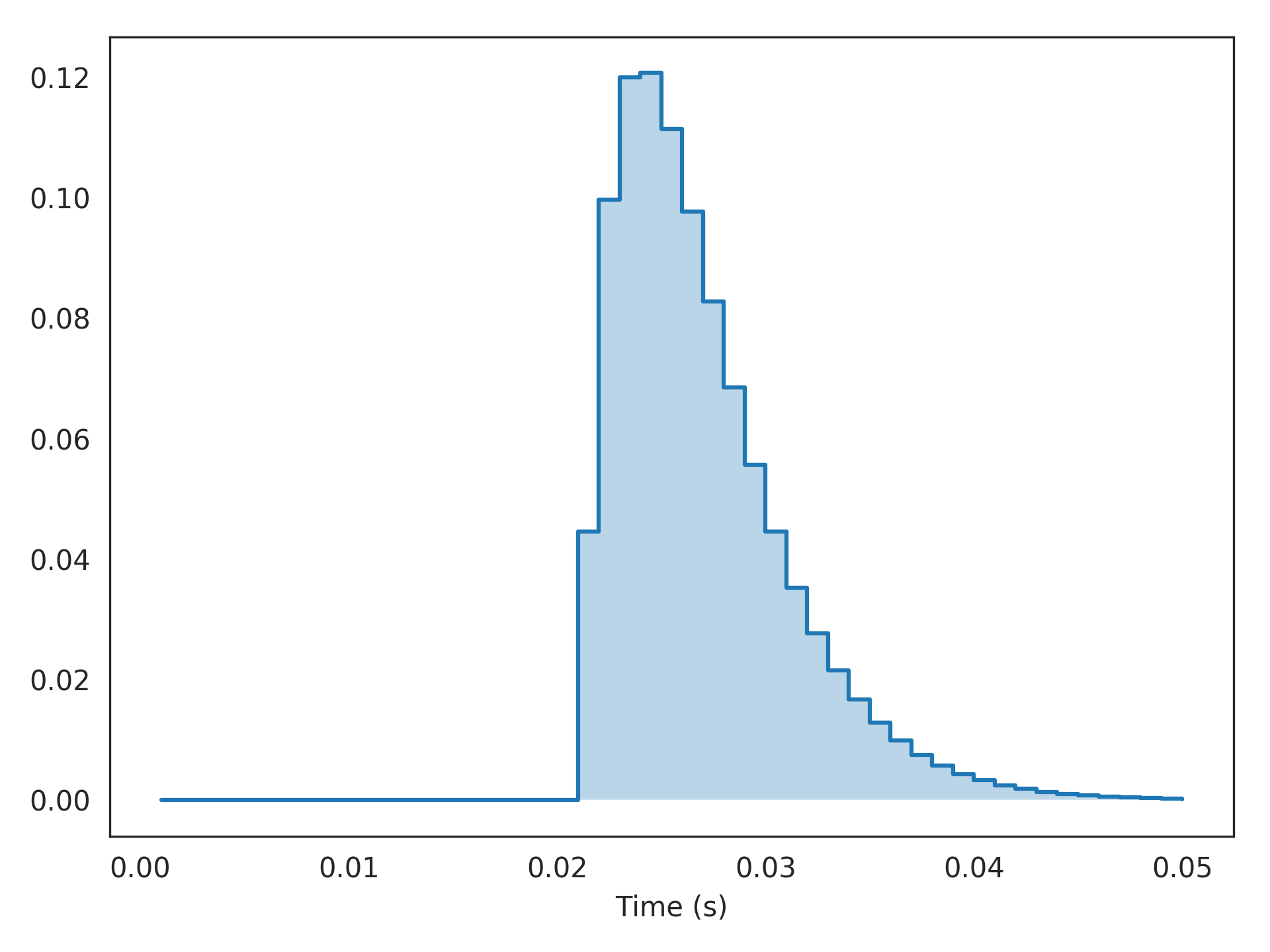# nengolib.signal.cont2discrete¶

nengolib.signal.cont2discrete(sys, dt, method='zoh', alpha=None)[source]

Convert linear system from continuous to discrete time-domain.

This is a wrapper around scipy.signal.cont2discrete(), with the same interface (apart from the type of the first parameter).

Parameters: sys : linear_system_like Linear system representation. dt : float Time-step for discrete simulation of target system. method : string, optional Method of discretization. Defaults to zero-order hold discretization ('zoh'), which assumes that the input signal is held constant over each discrete time-step.  alpha : float or None, optional Weighting parameter for use with method='gbt'. discrete_sys : LinearSystem Discretized linear system (analog=False).

Notes

Discretization is often performed automatically whenever needed; usually it is unnecessary to deal with this routine directly. One exception is when combining systems across domains (see example).

References

Examples

Simulating an alpha synapse with a pure transmission delay:

>>> from nengolib.signal import z, cont2discrete
>>> from nengolib import Alpha
>>> sys = Alpha(0.003)
>>> dsys = z**(-20) * cont2discrete(sys, dt=sys.default_dt)
>>> y = dsys.impulse(50)

>>> assert np.allclose(np.sum(y), 1, atol=1e-3)
>>> t = dsys.ntrange(len(y))

>>> import matplotlib.pyplot as plt
>>> plt.step(t, y, where='post')
>>> plt.fill_between(t, np.zeros_like(y), y, step='post', alpha=.3)
>>> plt.xlabel("Time (s)")
>>> plt.show()